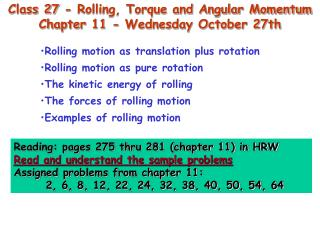Download PresentationClass 27 - Rolling, Torque and Angular Momentum Chapter 11 - Wednesday October 27th

Loading in 2 Seconds...

# Class 27 - Rolling, Torque and Angular Momentum Chapter 11 - Wednesday October 27th - PowerPoint PPT Presentation

Class 27 - Rolling, Torque and Angular Momentum Chapter 11 - Wednesday October 27th. Rolling motion as translation plus rotation Rolling motion as pure rotation The kinetic energy of rolling The forces of rolling motion Examples of rolling motion.I am the owner, or an agent authorized to act on behalf of the owner, of the copyrighted work described.
Download Presentation## Class 27 - Rolling, Torque and Angular Momentum Chapter 11 - Wednesday October 27th

An Image/Link below is provided (as is) to download presentation

Download Policy: Content on the Website is provided to you AS IS for your information and personal use and may not be sold / licensed / shared on other websites without getting consent from its author.While downloading, if for some reason you are not able to download a presentation, the publisher may have deleted the file from their server.

- - - - - - - - - - - - - - - - - - - - - - - - - - E N D - - - - - - - - - - - - - - - - - - - - - - - - - -
Presentation Transcript
1. Class 27 - Rolling, Torque and Angular Momentum Chapter 11 - Wednesday October 27th • Rolling motion as translation plus rotation • Rolling motion as pure rotation • The kinetic energy of rolling • The forces of rolling motion • Examples of rolling motion Reading: pages 275 thru 281 (chapter 11) in HRW Read and understand the sample problems Assigned problems from chapter 11: 2, 6, 8, 12, 22, 24, 32, 38, 40, 50, 54, 64

2. Rolling motion as rotation and translation Another way to visualize the motion: The wheel moves with speed ds/dt

3. Rolling motion as rotation and translation The wheel moves with speed ds/dt Another way to visualize the motion:

4. Rolling motion as pure rotation The kinetic energy of rolling

5. The forces of rolling (role of friction) • If the driving mechanism is a linear force, e.g. someone pushing the wheel through its center of mass, then the frictional force opposes the pushing force. • The sum of the two forces provide the linear acceleration. • Meanwhile, the friction force has a moment arm about the c.o.m., i.e. it provides a torque which, in part, causes the angular acceleration. • If the c.o.m. moves with constant velocity, and the wheel rotates with constant angular speed w = vcom/R, then there are no net forces on the wheel. • If however, the wheel has an angular acceleration, then there must be a frictional force at P in order for the wheel to move.

6. The forces of rolling (role of friction) • If the c.o.m. moves with constant velocity, and the wheel rotates with constant angular speed w = vcom/R, then there are no net forces on the wheel. • If however, the wheel has an angular acceleration, then there must be a frictional force at P in order for the wheel to move. • If the driving mechanism is a linear force, e.g. someone pushing the wheel through its center of mass, then the frictional force opposes the pushing force. • The sum of the two forces provide the linear acceleration. • One can also attribute the angular acceleration to the fact that Fpush has a moment arm about the point where the wheel makes contact with the road.

7. The forces of rolling (role of friction) • If the c.o.m. moves with constant velocity, and the wheel rotates with constant angular speed w = vcom/R, then there are no net forces on the wheel. • If however, the wheel has an angular acceleration, then there must be a frictional force at P in order for the wheel to move. • If, on the other hand, the driving mechanism is rotational, i.e. due to the torque of a motor, then the frictional force is in the opposite direction, and it causes the linear acceleration.

8. Rolling down a ramp • The frictional force is essential for the rolling motion. • If one analyzes the motion about the center of the disk, fs is the only force with a moment arm.

9. Rolling down a ramp Use: torque = Ia Thus: • However, we do not really have to compute fs (see section 12-3). • We can, instead, analyze the motion about P, in which case, Fgsinq is the only force component with a moment arm about P.

10. Some rotational inertia

11. More on rolling • Mechanical energy is NOT lost as a result of frictional forces. • It is, instead, converted to rotational kinetic energy. • This rotational kinetic is mechanical, so it may be converted back to translational kinetic energy, or gravitational potential energy. U U K = Kt + Kr This is the same as for the frictionless incline (acom = g sinq) with the additional Icom/MR2 term in the denominator.

12. More on rolling • The Yo-yo is essentially the same as the disc rolling down the incline, except that the string plays the role of the slope and the tension in the string plays the role of the friction. • Thus, q= 90o, and the relevant radius is Ro. • However, one should use R when calculating Icom.